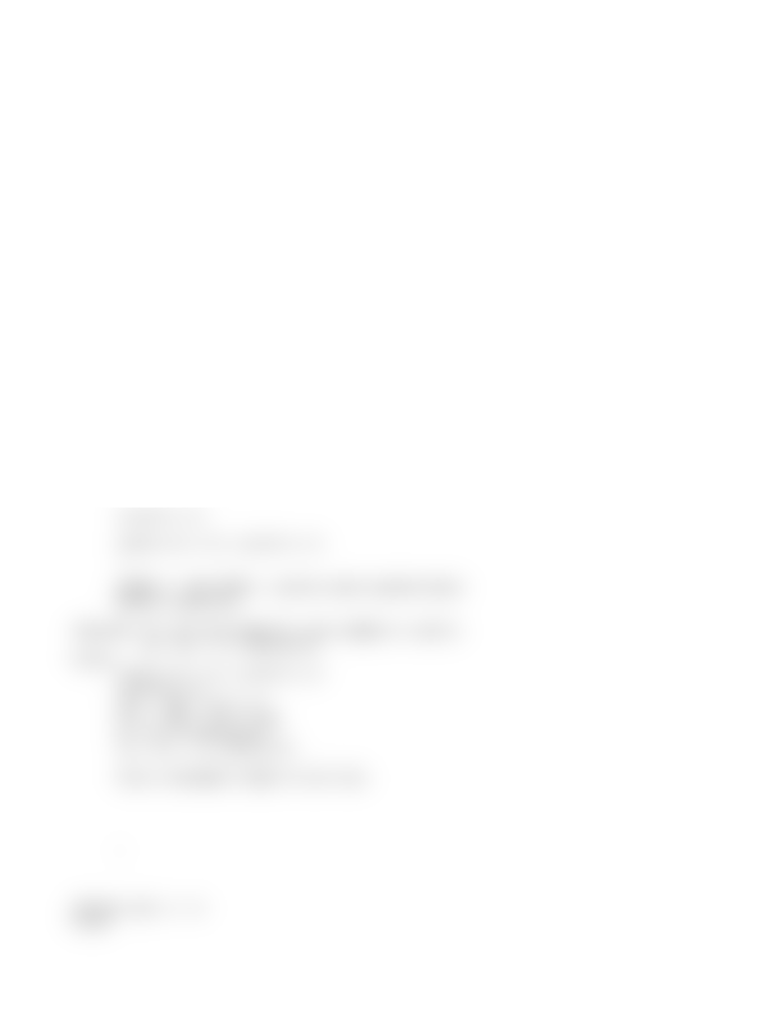# 15 - Induction Practice.txt

48 views2 pages
School
Course
Professor

For unlimited access to Class Notes, a Class+ subscription is required.October 28 2014
ETLC 2 - 001
More Induction
Remember to make explianation when substituting in a term during
the inductive step
Assume IH: sum(0 -> n, 3^i) = (3^n+1 - 1) / 2
Now, sum(0 -> n+1, 3^i) = sum(0 -> n, 3^i) + 3^(n+1) *** By definition of sum
***
= (3^n+1 - 1) / 2 + 3^(n+1) *** By IH ***
= (3^n+1 - 1) / 2 + 2*3^(n+1)/2 *** Multiply second term by 2/2 ***
= (3)((3^n+1) - 1) / 2 *** Collect n^(n+1) terms ***
= (3^(n+2) - 1) / 2
= (3^((n+1) + 1) - 1) / 2
Theorem: For all non-negative real numbers a and b,
(a + b) / 2 ≥ sqrt(a b)
Proof:
(a + b) / 2 ≥ sqrt(a b)
a + b ≥ 2 sqrt(a b)⋅ ⋅
a^2 + 2ab + b^2 ≥ 4ab
a^2 - 2ab + b^2 ≥ 0
(a-b)^2 ≥ 0
since a-b , (a-b)^2 ≥ 0∈ ℝ
WRONG!!! BAD PROOF. (pretty much upside down)
Better would be:
Theorem: For all non-negative real numbers a and b,
(a + b) / 2 ≥ sqrt(a b)
Proof:
since a-b , (a-b)^2 ≥ 0∈ ℝ
(a-b)^2 ≥ 0
a^2 - 2ab + b^2 ≥ 0
a^2 + 2ab + b^2 ≥ 4ab
a + b ≥ 2 sqrt(a b)⋅ ⋅
(a + b) / 2 ≥ sqrt(a b)
Every statement needs to be true.
Therom: Not 1 = 0
Proof
Unlock document

This preview shows half of the first page of the document.
Unlock all 2 pages and 3 million more documents.

Already have an account? Log in

# Get access

Grade+
\$10 USD/m
Billed \$120 USD annually
Homework Help
Class Notes
Textbook Notes
40 Verified Answers
Study Guides
1 Booster Class
Class+
\$8 USD/m
Billed \$96 USD annually
Homework Help
Class Notes
Textbook Notes
30 Verified Answers
Study Guides
1 Booster Class